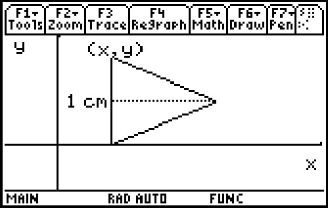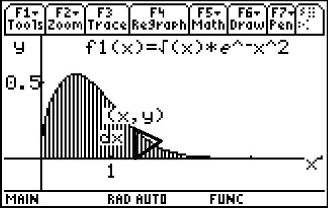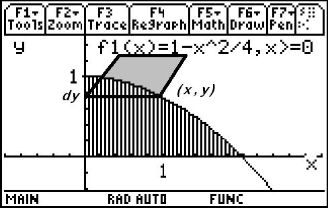# Activities

••• ##### Subject Area

• Math: Calculus: Applications of Integral, Antiderivatives and Definite Integrals
• Math: Calculus: Antiderivatives and Slope Fields

• ##### Author9-12

15 Minutes

• ##### Device
• TI-89 / TI-89 Titanium
• ##### Report an Issue

Volume by Cross Sections#### Activity Overview

Students explore the concept of finding the volume of a solid formed by cross sections of a function that form certain shapes.

#### Key Steps

•Students will be introduced to the concept of finding the volume of a solid formed by cross sections of a function that form certain shapes.

Since volume is the area of the base times the height and dV = Area ∙ dx, students review areas of various shapes like squares, semicircles and equilateral triangles.

•Calculator screen snapshots are used to help students get a visual of the volume under consideration.

•Students will practice what they learn with exam-like questions for homework or as an extension.